# RD Sharma Class 10 and Chapter Coordinate Geometry Maths Solutions

## Maths Class 10 and Chapter Coordinate Geometry RD Sharma SolutionsFilter
Filters :
CLASSES
•
•
•
•
•
•
•
CHAPTERS
•
• 6 More

### RD SHARMA Class 10 | COORDINATE GEOMETRY

The coordinates of one end point of a diameter of a circle are (4,-1) and the coordinates of the centre of the circle are (1,-3) . Find the coordinates of the other end of the diameter.In chapter 13, we have learned about Probability. In this chapter, we will focus on Coordinate Geometry. Getting the right information on how to find the coordinates in an accurate manner shall be discussed here. Also, we will concentrate on the area of a triangle that would make you get the perfect knowledge on how to calculate in the best way. In Geometry, students should be able to get all the essential information on how to work on different problems without any worry at all. Doubtnut has made things much easier in providing with the best exercises and examples as well.

NCERT books do not prove to be much useful for the students.  This is because it helps in clearing the essential information which does not lead to scoring good marks in the exams. Unless students get the right idea on how to tackle different questions most efficiently, it would not be possible to achieve success at all. By not being able to score good marks, students look forward to the different guides and reference books. Here, Class 10 Maths RD Sharma Solutions proves to be of much use for the students. They get some good idea on how to tackle different questions without any problem at all.

As already discussed, that the NCERT math book is not sufficient for the students to crack the exams, a team of expert tutors of Doubtnut has made it possible to make things much easier for the students. They have come up with the best and useful online study materials that make students get the perfect idea on how to tackle different questions most easily. It has made things much interesting for them where they can get to practice different exercises online.

## Topics and subtopics of RD Sharma class 10 Chapter 14 Coordinate Geometry

### Finding the coordinates

It is possible to find the coordinates of two equilateral triangles in the best way.  Well, let us have a good look at some of the exercises below.

Exercise 1:  Two equilateral triangles PQR and PQR' has the base PQ with side 2a lies along y-axis such that the mid-point of PQ is at the origin. Can you find out the coordinates of the vertices R and R' of the triangles?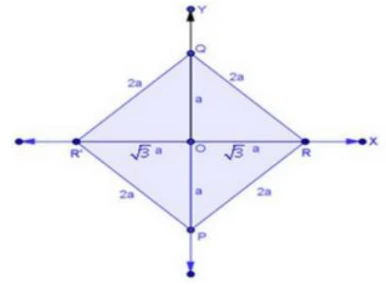Solution:

We have two equilateral triangle PQR and PQR' with side 2a.

O is the mid-point of PQ.

In Q0R. ∠QOR = 90°

Hence, by Pythagoras theorem

OR2 + OQ2 = QR2

OR2 = (2a)2 – (a)2

OR2 = 3a2

OR = √(3) a

Coordinates of vertex R is (√3a, 0) and coordinate of vertex R' is (- √3a, 0)

Exercise 2:  Can you find out the distance between the following pair of points?

1. (- 6, 7) and (-1, -5)
2. (a + b, b + c) and (a -b, c - b)
3. (a sin α, - b cos α) and (- a cos α, b sin α)
4.  (a, 0) and (0, b)

Solution:

(i) We have P (- 6, 7) and Q (- 1, - 5)

Here,

x1 = - 6, y1 = 7 and

x2 = -1, Y2 = - 5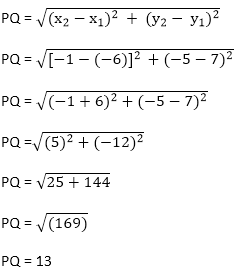(ii) We have P (a + b, b + c) and Q (a - b, c - b)

Here, x1 = a + b, y1 = b + c and x2 = a - b, Y2 = c – b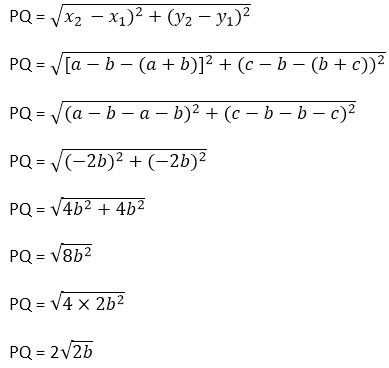(iii) We have P(a sinα, – b cos α) and Q(-a cos α, b sin α)

Here, x1 = a sin α, y1 = - b cos α and

x2 – a cos α, y2 = b sin α(iv) We have P (a, 0) and Q (0, b)

Here, x1 = a, y1 = 0, x2 = 0, y2 = b,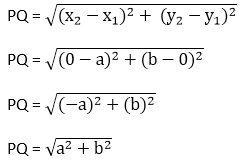### Area of a triangle

You know how to calculate the area of a triangle when its base and corresponding height are given.

Area of a triangle = 1/2x base x altitude

We will have a look at the exercise below to get the right idea:

Exercise 1: Find the area of a triangles whose vertices are

(i) (6, 3), (-3, 5) and (4, - 2)

(ii) [(at12, at1),( at22, 2at2)( at32, 2at3)]

(iii) (a, c + a), (a, c) and (-a, c - a)

Solution:

(i) Area of a triangle is given by

1/2[x1(y2 - y3) + x2(y3 - y1) + x3(y1 + y2)]

Here, x1 = 6,y1 = 3, x2 = -3, y2 = 5, x3 = 4,y3 = -2]

Let A(6, 3), B(-3, 5) and C(4,-2) be the given points

Area of ABC = 1/2 [6(5+2)+(-3)(- 2 -3)+ 4(3 - 5)]

=1/2 [6 × 7- 3 × ( – 5) + 4( – 2)]

= 1/2[42 +15 - 8]

= 49/2 sq. units

(ii) Let A = (x1, y­1) = (at12, 2at1)

B = (x2,y2) = (at22, 2at2)

= (x3, y3) = (at32, 2at2) be the given points.

The area of ABC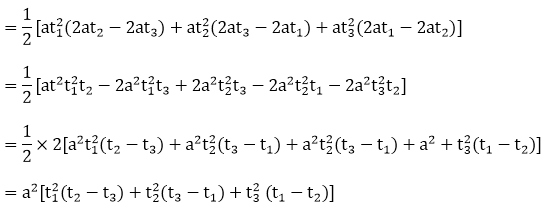(iii) Let A = (x1,y1) = (a, c + a)

B = (x2, y2) = (a, c)

C = (x3, y3) = (- a, c - a) be the given points

The area of ABC

= 1/2[a ( – {c - a}) + a(c – a - (c + a)) +( - a)(c + a - a)]

= 1/2 [a(c – c + a) + a(c – a – c - a) - a(c + a - c)]

= 1/2[a × a + ax( - 2a) – a × a]

= 1/2[a2 - 2a2 - a2]

= 1/2×(-2a)2

= - a2

Exercise 2: Find the area of the quadrilaterals, the coordinates of whose vertices are

(i) (-3, 2), (5, 4), (7, -6) and (-5,- 4)

(ii) (1, 2), (6, 2), (5, 3) and (3, 4)

(iii) (-4, -2), (-3, -5), (3, -2), (2, 3)

Solution:

(i) Let A(-3, 2), B(5, 4), C(7,- 6) and D ( -5, – 4) be the given points.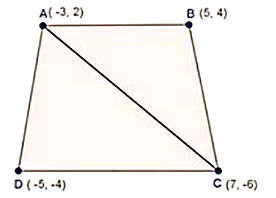Area of ABC

= 1/2[-3(4 + 6) + 5(- 6 - 2) + 7(2 - 4)]

= 1/2[-3×1 + 5×(-8) + 7(-2)]

= 1/2[- 30 – 40 -14]

=  - 42

But area cannot be negative

∴  Area of ADC = 42 square units

= 1/2[-3( – 6 + 4) + 7(- 4 - 2) + (- 5)(2 + 6)]

= 1/2[- 3( – 2) + 7(- 6) – 5 × 8]

= 1/2[6 – 42 - 40]

= 1/2 × – 76

= - 38

But area cannot be negative

∴ Area of ADC = 38 square units

= Ar. of ABC+ Ar of ADC

= (42 + 38)

= 80 square. Units

(ii)  Let A (1, 2) , B (6, 2) , C (5, 3) and (3, 4) be the given points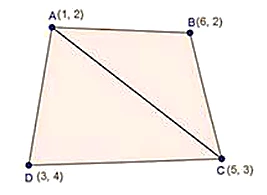Area of ABC

= 1/2[1(2 - 3) + 6(3 - 2) + 5(2 - 2)]

= 1/2[ -1 + 6 × (1) + 0]

= 1/2[ – 1 + 6]

= 5/2

= 1/2[1(3 - 4) + 5(4 - 2) + 3(2 - 3)]

= 1/2[-1 × 5 × 2 + 3(-1)]

= 1/2[-1 + 10 - 3]

= 1/2

= 3

= Area of ABC + Area of ADC(iii) Let A (- 4, 2), B( – 3, – 5), C (3,- 2) and D(2, 3) be the given points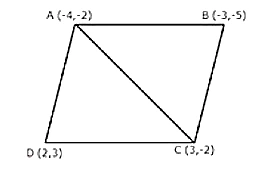Area of ABC = 1/2|(- 4)(- 5 + 2) - 3(-2 + 2) + 3(- 2 + 5)|

= 1/2|(-4)(-3) – 3(0) + 3(3)|

= 21/2

Area of ACD = 1/2|( – 4)(3 + 2) + 2( – 2 + 2) + 3( – 2 – 3)|

= 1/2|- 4(5) + 2(0) + 3(- 5)|= (- 35)/2

But area can’t be negative, hence area of ADC = 35/2

Area (quadrilateral ABCD) = 21/2 + 35/2